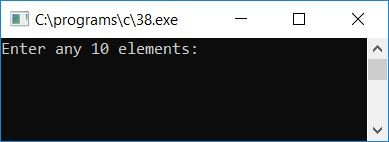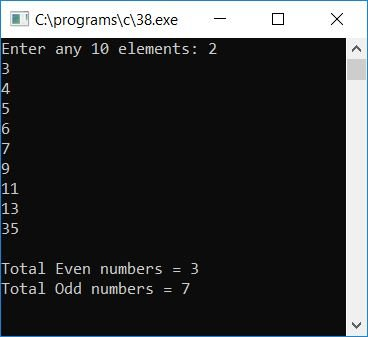# C Program to Count Even and Odd Numbers in an Array

In this tutorial, we will learn about how to create a program in C that will read few elements say 10 elements (numbers) from user (at run-time) as input and count all the even and odd numbers or elements present inside the given 10 array elements to print it as output. Here is the program:

```// Write a program in C to read 10 Elements
// in an array and count all even and odd numbers
// -----------codescracker.com-----------

#include<stdio.h>
#include<conio.h>
int main()
{
int arr, evenCount=0, oddCount=0, i;
printf("Enter any 10 elements: ");
for(i=0; i<10; i++)
{
scanf("%d", &arr[i]);
}
for(i=0; i<10; i++)
{
if(arr[i]%2==0)
evenCount++;
else
oddCount++;
}
printf("\nTotal Even numbers = %d", evenCount);
printf("\nTotal Odd numbers = %d", oddCount);
getch();
return 0;
}```

As the program was written under Code::Blocks IDE, therefore after sucessful build and run, here is the first snapshot of the sample run:Supply any 10 array elements (numbers) and then press `ENTER` key to count and print the total number of even and odd numbers among all the given 10 numbers as shown in the second snapshot of the sample run given below:Here are some of the main steps used in above program:

• Receive any 10 numbers (10 elements for the array) as input
• Create a for loop that runs 10 times, starts from 0 to 9 as indexing in array starts with 0
• Inside the for loop, check whether the current element (number) is an even number or an odd number
• If it is an even number, then increment the evenCount variable otherwise increment the oddCount variable
• Here evenCount represents the total number of even number and oddCount represents the total number of odd number
• Never forgot to initialize these two variables with 0 before entering into the for loop to check and increment the corrensponding variable's value by one each time
• After checking for each and every element of the array, come out from the loop and print the value of both the variable

Tools
Calculator